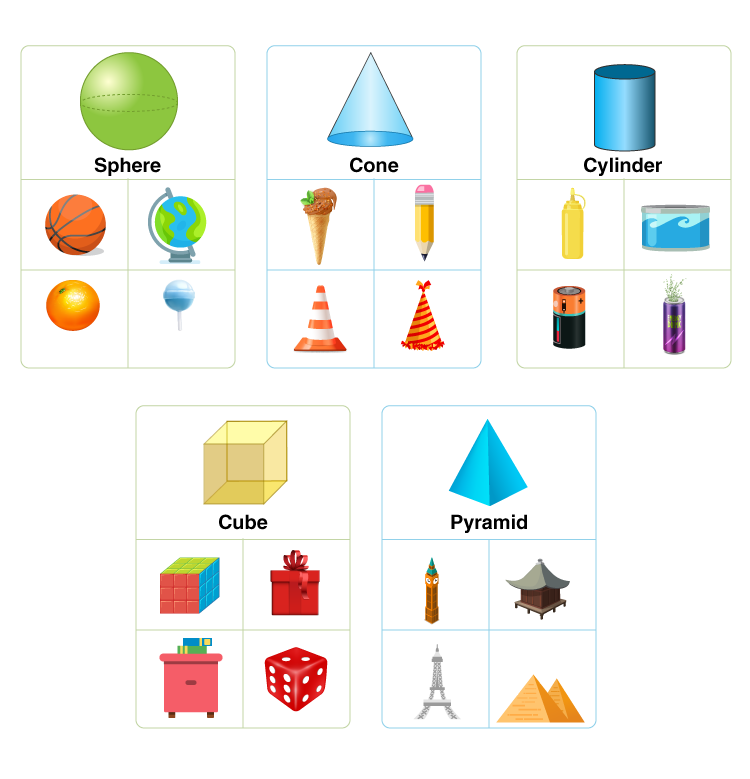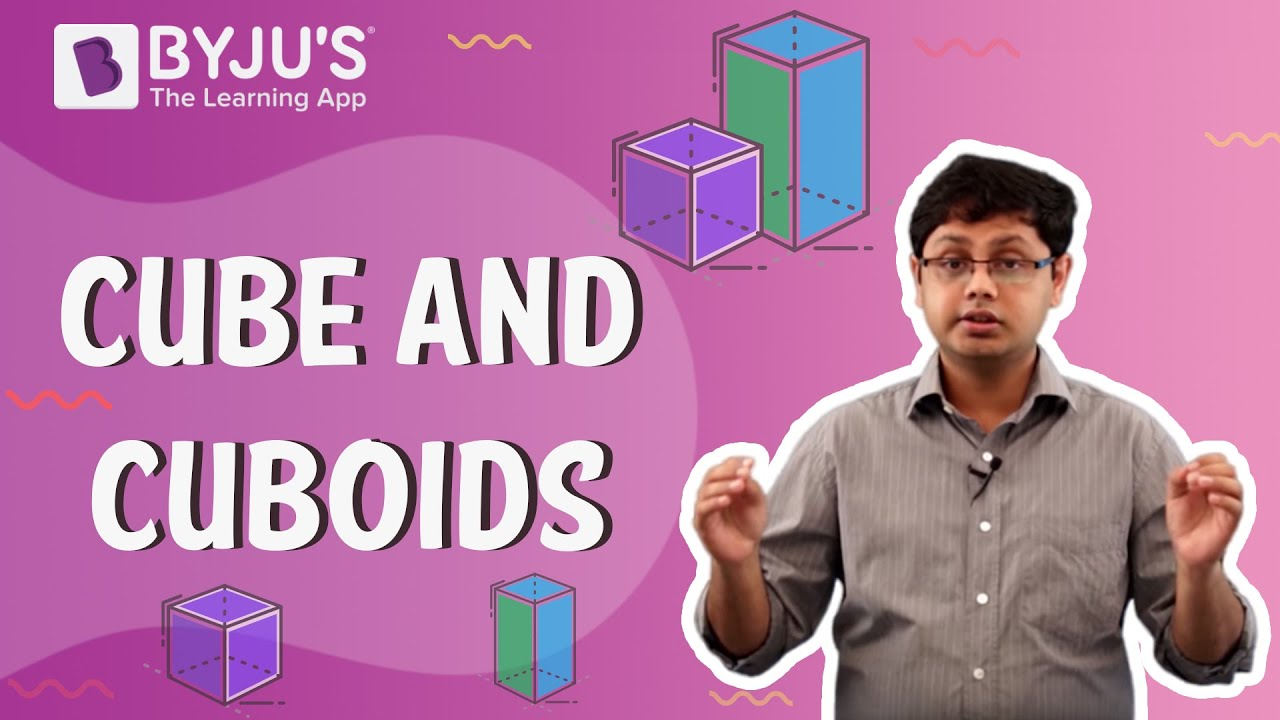# Examples for Cone, Sphere, Cylinder and Cuboid

Cone, sphere, cylinder and cuboid are the three-dimensional solids which have many real-life examples. All these shapes help us to identify the shape of the object in the real world based on the dimensions given. For example, the shape of the bed is in the form of the cuboid.

Have you ever imagined the shape of the ice cream cone, or the shape of a football, or the shape of the cylinder? etc. All these shapes are 3d shapes which are aligned to three axes, such as x-axis, y-axis and z-axis. Let us see some of the examples here.## Real-Life Examples of Cone, Sphere, Cylinder and Cuboid

The definition of each shape with their real-life examples is given below:

### Examples of Cone

Cone can be defined as a pyramid having the circular cross-section. Examples are:

• Party hat
• Ice cream cone
• Funnel
• Traffic cone
• Waffle cone
• Megaphone
• Christmas tree

### Examples of Sphere

The sphere can be defined as a round solid figure whose distance from any point on its surface to the midpoint is the same or equal. Examples of sphere include:

• Ball
• Planets
• Moon
• Sun
• Eyeball
• Orange
• Marbles

### Examples of Cylinder

The cylinder can be defined as a solid geometrical figure with straight parallel sides and a circular or oval cross-section. Examples are:

• Pipes
• Cold drink cans
• Water tanks
• Battery
• Gas cylinder
• Candle
• Test tube
• Beaker
• Fire extinguisher

### Examples of Cuboid

A solid shaped figure which has six rectangular faces perpendicular to one another. It is a three-dimensional shape with length, breadth, and height. Examples include:

• The lunch box
• Bricks
• Shoebox
• Book
• Carton boxes
• Mattresses
• Cabinet
• Cubicles
• Fridge## Examples of Combination of Solids

The solid shapes could be combined with each other to produce a new shape. Suppose a capsule which is shaped like a cylinder is the combination of two hemispheres.

Let us see here some more examples:

• A tent is a combination of cone and cylinder shapes.
• An ice cream cone is a combination of cone and hemisphere shape ice-cream in it.
• Mushroom has a cone-shaped cap over a cylinder-shaped body.
• A house whose top is a cone shape and walls are constructed to form a cuboid shape.

Also, check: Volume Of A Combination Of Solids

4.5 (19)(51)(9)

#### Choose An Option That Best Describes Your Problem

Thank you. Your Feedback will Help us Serve you better.

1. Aravind

Nice examples

1. Shivani Harish

Yes , This helped my sister’s to visualise and study in deep all about solid shapes.

2. Nice

2. Rita Vella

Great thanks for this. Great help to simplify the examples of 3d shapes to the children.

3. Avni Kushwaha

4. Ishaani

Thank you

5. Pranshu

Thanks a lot

6. Nice examples super 😍😍

7. satish kumar